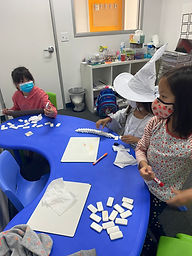## Ms. Hiral

### Target 1​

###### Lesson Type:

Continuation

Number Operation

:

Computation

Multiply within 100.

###### 1:

Understand that multiplication represents the combination of equal groups of objects.

###### 2:

Understand that the same quantity can be group in multiple ways, creating different multiplication equations.

###### 3:

Understand that multiplication equations can be written both vertically and horizontally and multiplication is often identified by an “x” in an equation.

3rd

###### Vocabulary:

Split Method, Multiplication

Activities:

Students played dominoe war, they had to multiply both sides of the dominoe and whoever had the highest product won.

Students were intriduced to the split method and worked through equations together on the whitebaord.

Students then rolled dice to create new multiplication equations for them to individually solve.### Home Exploration

###### Guiding Questions:## Absent Students:

### Target 2

:

###### Vocabulary:

Activities:### Home Exploration

###### Guiding Questions:### Target 3

:

###### Vocabulary:

Activities:### Home Exploration